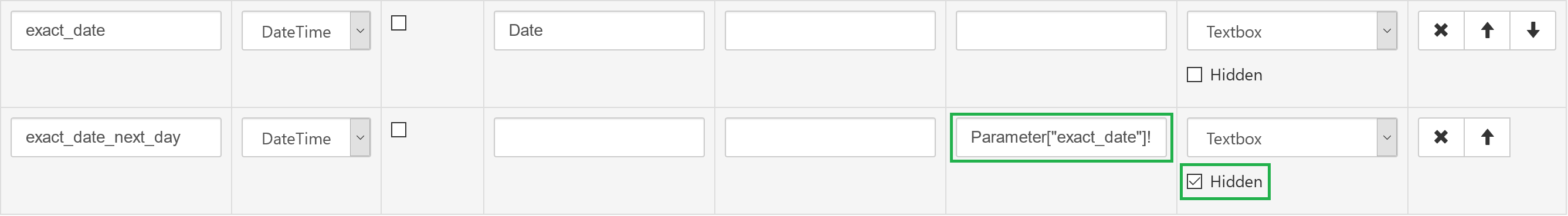# Date Range Filter Setup

Setup of report parameters for filtering by date might be a bit tricky, especially in case of timestamp fields because they cannot be filtered with simple equality comparison with a some date value. Let's discover how to do setup report parameters for typical cases.

### Start Date + End Date

This is simplest case when date range is specified with 2 separate user-entered report parameters:Application of these parameters in the data source query is rather trivial:

Select Query
```SELECT * FROM some_table WHERE 1=1 @start_date[ and date_column >= {0} ] @end_date[ and date_column < {0} ]
```
Filter JSON
```{ \$and: [
{"_id":{\$exists:true}},
@start_date[ {{ "date_field" : {{ \$gte: ISODate( {0} ) }}  }}, ]
@end_date[ {{ "date_field" : {{ \$lt: ISODate( {0} ) }}  }}, ]
] }```
Filter Expression
``` "1"="1" @start_date[ and "date_fld":field>="{0}":var ]  @end_date[ and "date_fld":field<"{0}":var ]
```
MDX Query Tokens

Ensure that your MDX template uses `@CUBE` token which is defined like this:

```SelectFromCube("YOUR_CUBE_NAME").Range("[Date].[Date]", date_start, date_end)
```

You may notice that start_date is inclusive (meaning is "starting from this date"), and end_date is exclusive (meaning is "everything before this date"); this guarantees correct filtering if dates have non-zero time part. If you want to make end_date inclusive you can specify the following Expression in the parameter configuration: `Parameter["end_date"]!=null ? Parameter["end_date"].AddDays(1) : null`.

Important: if your dates are stored as numbers (UNIX timestamp in seconds or milliseconds) your DateTime parameters should be converted to numbers with help of expressions.

### Exact Date

In some cases you might want to filter report data by exact date. For database 'Date' column (field) application of the date filter parameter is trivial, however in case of timestamps you'll need to introduce additional (hidden) parameter that calculates 'next day date':Expression for this parameter should be: `Parameter["exact_date"]!=null ? Parameter["exact_date"].AddDays(1) : null`. Application of these parameters in the data source query is exactly the same as in case of start/end parameters (described above) where exact_date corresponds to start date, and exact_date_next_day is an end date respectively.

### Pre-configured date ranges like today, yesterday, last 7 days, last 30 days etc

Another typical case for operational reports is filtering by custom pre-defined date ranges. For this purpose you need to configure the following report parameters:

• Parameter with dropdown editor that will be used by end-user to choose the range:• Parameter(s) that calculate start date (and end date, if used) by the range option choosen by end-user:Expression: `Parameter["date_range"]!=null ? Date.Now().Date.AddDays(-Parameter["date_range"]) : null`
Note that `Date.Now()` returns server's date/time. To make the calculation independent from server's timezone offset you may use `Date.UtcNow()` and with `.AddHours(offsetInHours)` get timestamp exactly in your timezone.
• In the data source query apply only date_range_start (and end date if you calculate it too) in the same way as in case of explicit start/end date (described above).

### Filtering on UNIX timestamps

To convert DateTime-type report parameters to numbers use the following expressions:

• When number represents seconds-since-the-epoch: `<date_value>.Subtract(Convert.ToDateTime("1970-01-01 00:00:00")).TotalSeconds`
• When number represents milliseconds-since-the-epoch: `<date_value>.Subtract(Convert.ToDateTime("1970-01-01 00:00:00")).TotalMilliseconds`

### Timezone offset handling

SeekTable doesn't handle timezones automatically on its side; this means that unmodified Date/Time value is used in the data query condition. From this perspective, the following situations are possible:

• timestamps in your DB are stored in local timezone, and this timezone is ok for reports. Nothing special is needed for filters where user enters concrete date. If you use SeekTable server time in expressions it is enough to add an offset of your local timezone to `Date.UtcNow()` result with `AddHours` method.
• timestamps in your DB are stored in UTC, and you want to view reports in context of some concrete timezone. You need to add an offset of this timezone to dates entered by the end-user by specifying Expression for these parameters: `Parameter["start_date"]!=null ? Parameter["start_date"].AddHours(offsetInHours) : null`.

Many timezones have daylight saving time shift, and if you don't want to change the constant in expressions twice per year you can use `Date.TimezoneUtcOffsetHours("linux_timezone_name")` function (see list of TZ names). For example, `Date.TimezoneUtcOffsetHours("CET")` returns `2` in daylight saving period and `1` otherwise.